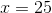## Example Questions

### Example Question #7 : Polynomials

Simplify: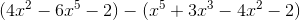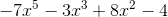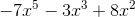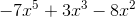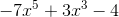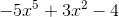Explanation:

When subtracting polynomials, it's helpful to remember that the "minus sign" gets distributed. It's as if the two polynomials are being added and a -1 is in front of the second polynomial.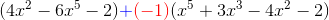This -1 will get multiplied to all the terms in the second polynomial that is being subtracted from the first, so it becomes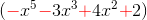. You may note that by multiplying by -1, every term in the polynomial switches its original sign. The problem then becomes: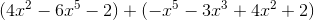From here, in order to simplify, because there's no equal sign, it can be deduced that we are not working toward a solution for x. The original problem is presented as an expression so an expression as an answer will be expected. In order to work towards a final simplified expression, like terms must be collected. This will provide the final answer.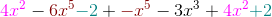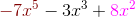### Example Question #11 : Polynomials

Simplify: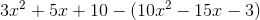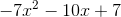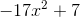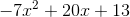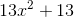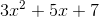Explanation:

Begin by distributing the subtraction of the second term in this question: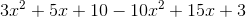Now, you merely need to combine like terms: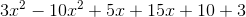### Example Question #12 : Polynomials

If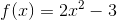, then what does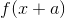equal?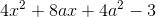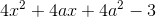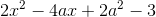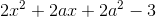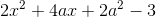Explanation:

To solve this equation, we substitute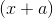in for every instance ofseen in the original equation.

Therefore the new equation would read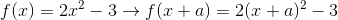Now we must square the expression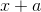. To do this, you must multiply the expression by itself. Therefore: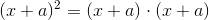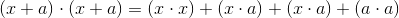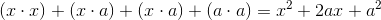We must now plug in our new value for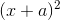into our original equation in place of.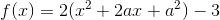Now we must distribute theinto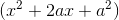. To do this, you multiply each expression within the parenthesis by: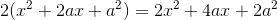Therefore, our answer is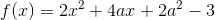.

### Example Question #13 : Polynomials

The expression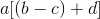is equivalent to which of the following?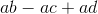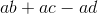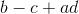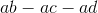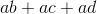Explanation:

To answer this question, we must distribute theto the rest of the variables,, andthat are within the brackets.

To distribute a variable or number, you multiply that value with every other value within the brackets or parentheses. So, for this data: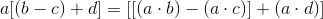We then simplify the expression by combining the variables we are multiplying together into expressions. For this data: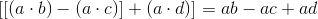Be sure to keep all of your operations the same within the problem itself, unless the number being distributed is negative, which will then switch the signs with the brackets from positive to negative or negative to positive.

Therefore, our answer is.

### Example Question #14 : Polynomials

Solve the equation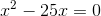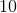Explanation:

To answer this question, we are solving for the values ofthat make this equation true.

To this, we need to geton a side by itself so we can evaluate it. To do this, we first add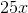to both sides so that we can then begin to deal with thevalue. So, for this data: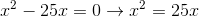can also be written as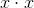. Therefore: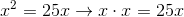Now we can divide both sides byand find the value of.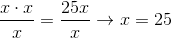Therefore, the answer to this question is